☜ Navigate through the Golden Ratio pages ☞

# Calculating The Golden Ratio

Using the quadratic equation and continued fractions to find a value for the golden ratio. There are no animations here, but the next two pages have interactive tools.

## Brief Explanation

So what is the value of the golden ratio? It is often represented by the greek letter φ (phi). What is φ? On this page we look at two ways to answer this question. One leads to a decimal value, the other to a continued fraction.

We start out with this diagram, and then use the fact that the two golden rectangles are similar — in the full geometric sense: the ratios of length to width are the same.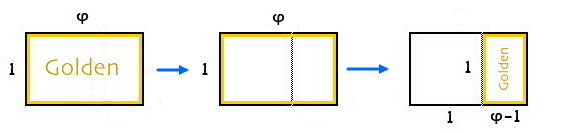## The Decimal Value

This illustration shows which ratios are supposed to be equal.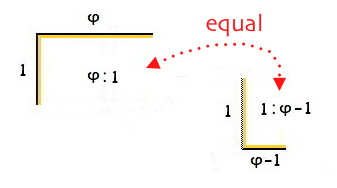Turning that into an equation give us: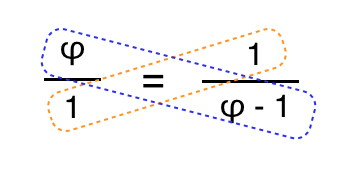When you have equal ratios, the two diagonal products are equal, so we get

φ2 - φ = 1

Now, subtract 1 from both sides, giving

φ2 - φ - 1 = 0

and solve using the well-known quadratic formula. We will assume you are familiar with using that tool, and just present the answer.

Recall that the quadratic equation gives two solutions, and sometimes you have to figure out which one really applies to your situation. In this case, one of the solutions is negative, so we ignore it. The positive solution is:

φ = (1 + √5)/2 ≈ 1.6180339...

The first thing we notice about φ is that it is irrational, because it has the square root of five in it. It is notable that this number, which is all about ratios, is itself not rational. The next two pages will investigate this topic further.

## The Continued Fraction

There is another way we can approach finding an expression for φ. In a way, we do everything “backwards” from the solution shown above. First, we rewrite the ratio in a different — but still correct — way, by flipping the fractions and switching left and right, as:Adding 1 to both sides gives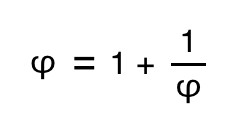Now we do something that is the opposite of the way we normally reduce equations. We expand it instead, using the following substitution:That yields the following equation: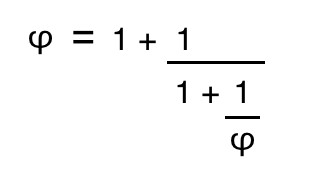It looks like we are losing ground instead of gaining it! That is, the expression is getting more complicated. But if we persevere — in other words, continue making the same substitution — we come up with an infinite expression called a continued fraction: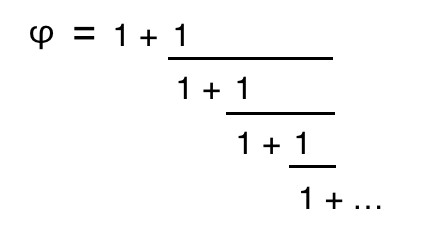## Remarks

The next page in this sequence will explore more about how to interpret expressions like this. Just looking at it can give you an idea of how the golden ratio is connected to such topics as recursion and fractals.

☜ Navigate through the Golden Ratio pages ☞